Tuesday, 25 February 2020# Kriging – Do You Know What Samples are Being Used?

Introduction

In the late 1970s to early 1980s Ordinary Kriging (OK) became the block grade estimation method of choice. This was mainly due to it being an unbiased estimation method that attempts to minimize the extension (estimation) variance of the estimate. Since its inception the way resource modellers use OK has evolved, with increased user experience and improvements in technology, allowing us to significantly vary the input parameters. This article examines the positive and negative evolution in the use of the OK estimation method discussing changes in composite length, number of samples, declustering, octant search, and negative weights.

All examples are generated using constant variogram parameters and search ellipses.

De-clustering

The unbiased nature of OK is demonstrated in Figure 1, where OK weights are compared to inverse distance squared weights (ID2).

The two samples at the top of Figure 1 are in close proximity to each other. This results in their allocated OK weights (coloured red) being almost half that of the weight allocated to them as ID2 samples (coloured black). OK declusters the samples resulting in the lower weights for the two closely spaced samples. Inverse distance gives all samples the same weight, biasing the estimated block grade.

Figure 1 Comparison between Kriging and ID2 weights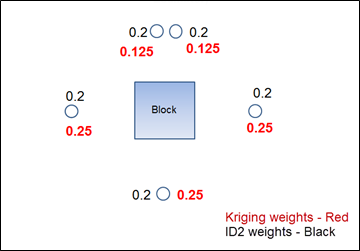Due to the declustering of samples when applying sample weights, OK provides a better estimate of block grades than ID2.

Drillhole Sample Weighting

In the late 1980s to early 1990s some resource modellers began compositing drillhole samples to very large lengths. Samples assayed on one metre lengths were being composited to ten metre

lengths or even greater. The initial reaction was “these people were smearing the grade and losing the ability to provide local estimates”.

One of the reasons for compositing to large lengths is to mitigate the way sample weights are applied by OK as a result of its declustering of sample data. Bearing in mind that declustering of data is one of the aspects of OK that makes it so appealing.

Figure 2 shows an example of sample weights applied to intervals down a single drillhole during OK.

Figure 2 Kriging weights for ten samples from one drillhole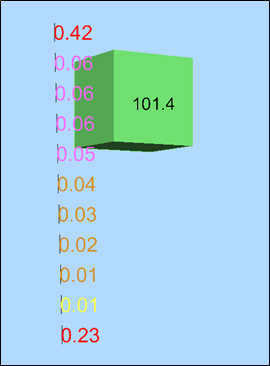Note Green box is the block being estimated and the black number is the average block grade. The values assigned to the drillhole intervals are the kriging weights applied to one drillhole.

In this example the block grade has been estimated using OK with discretization at a 3 m spacing along each axis. The two end samples have the greatest weights which total approximately 65% of the total weighting. It does not matter how many samples from one drillhole are used, the two end samples will always have the greatest weight and the samples in between will have very low weights.

The samples in the middle of the drillhole are the closest to the block and should therefore have the greatest weights. The discrepancy in sample weighting is due to OK declustering the sample data. Samples in the middle have samples either side while the end samples only have one close sample and are therefore considered less biased, hence a higher weighting. This would suggest that the use of two samples only per drillhole is the preferred sample number, hence the use of composites of greater lengths. Compositing samples to longer lengths whilst still using two samples per drillhole will give a better global block grade estimate.

The samples at the end of each drillhole will have a high percentage of the total weights.

Maximum Number of Samples

Following the 1980s and 1990s trend of using large composites, the use of kriging neighbourhood analysis (KNA) became the norm in the early 2000s. The value calculated for the “slope of regression” (based on the kriging variance), was used to select the number of samples needed to estimate a block grade. A slope of regression value approaching one was considered necessary. As the number of samples used in the estimate increased, the estimate of the slope of regression also increased. This resulted in the number of samples used per estimate increasing to amounts as high as 60 or even more. This was partially justified by the use of estimation software offering octant searches and the ability to set a maximum number of samples sourced from a single drillhole. KNA also considered minimizing the number of negative weights.

Figure 3 shows an example of using 60 samples to estimate the block grade with a maximum of six samples per drillhole and using octant search (shown using Datamine software).

Figure 3 Kriging weights 60 samples six samples per drillhole with octant search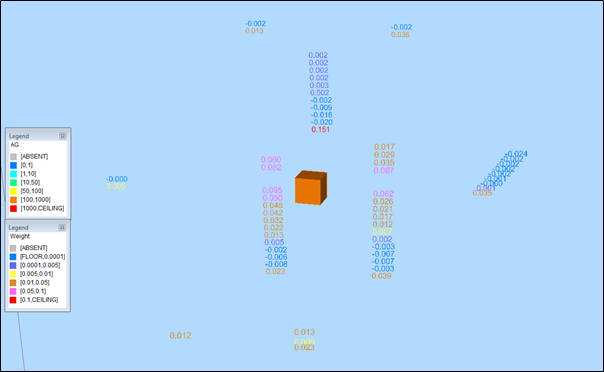Note: The numbers are the Kriging weights and are coloured by the weight value. The block is coloured by the silver grade.

Figure 3 shows that the samples selected at each end of the drillhole have the largest weights. When a drillhole has an increase in the number of samples included in the estimate from using two or more octants, the samples in the middle continue to have very low or even negative weights. Here eight samples have a total kriging weight of approximately 62 % out of the 60 samples used in the estimate. A total of 22 samples had negative weights. If the negative weights were not allowed, the negative weights would then be set to zero, resulting in a total of 22 samples with zero weights.

Since the early 2000s the number of samples used per estimate has been reduced, as estimators seek to provide local block grade estimates using local sample data and not global estimates.

The use of a large number of samples per drillhole even when using octant search results in the samples in the middle of each drillhole (closest to the block being estimated) having very low or even negative weights.

What Estimation Parameters Do I Use?

When deciding on what estimation parameters to use, some of the questions you should ask are:

• How many samples should be used to estimate a block grade?
• How many samples should be used from the same drillhole?
• Should octant searching be used?
• Should negative weights be allowed?
• What to do with twinned drillholes?

In order to answer these questions, a review of the impact of changing the maximum number of samples and maximum number of samples per drillhole, with and without octant search was carried out. The same sample data set was used as displayed in the previous examples. This is different to a KNA as the slope of regression, search ellipse, orientation and or ranges were not considered.

Results

Number of samples: the number of samples used in the estimate did have an impact on the estimated block grade where a maximum number of samples per drillhole is not used. Table 1 lists the estimated block grade for a series of maximum number of samples, without limiting the number of samples from a single drillhole.

Table 1 Estimated block grade versus number of samples

 No. Samples Average grade 60 0.19 50 0.20 40 0.17 30 0.18 20 0.19 10 0.36 5 0.68

Table 1 shows very similar block grades when using 20 to 60 samples. In this example, the grade increased where there were less samples. The block estimated was close to one hole, resulting in all 60 samples coming from the one hole.

Figure 4 shows the sample grades and weights when using 60, 20 and 5 samples per estimate, sorted by the closest sample. This figure shows that when using 60 and 20 samples the closest sample (high grade in this case) received approximately the same weight, whereas for 5 samples the first sample received a much higher weight hence the increase in block grade. The figure also shows the samples furthest away from the block received the largest weight.

Figure 4 Estimated grade versus number of samples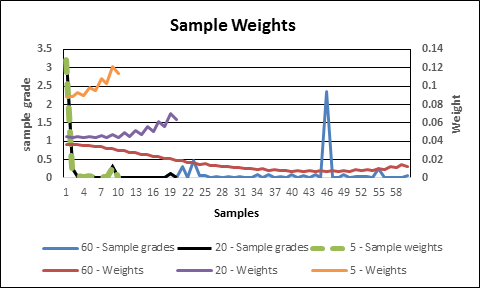Number of samples from a drillhole: The number of samples used per hole has a big impact on the estimated block grade. Table 2 show the results of the estimated block grades, based on different maximum numbers of samples, where a maximum of three samples per drillhole is used. This table shows that even though the number of samples and number of drillholes used increases, the block grade does not significantly change.

Table 2 Estimated block grade using different number of samples with a maximum of three samples per hole

 No. samples Block grade No.holes No. of larger weights Largest weight No. negative weights 60 0.87 20 3 0.214 2 50 0.79 17 3 0.214 0 40 0.79 14 3 0.216 0 30 0.78 10 3 0.216 0 20 0.77 7 3 0.218 0 10 0.79 4 3 0.227 0

The field “No. of Larger Weights” in Table 2 lists the number of larger sample weights.

Figure 5 shows the weights and distance to block, sorted by the sample distance to the block. As this block estimate is based on a minimum of 50 samples with a maximum of 10 from a single hole, the closest 10 samples have a much larger weight than the remaining 40. Also, three samples have even larger weights.

Figure 5 Kriging weights with distance from block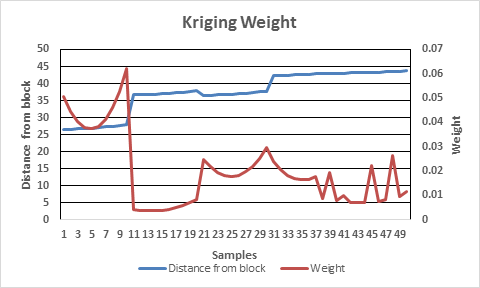Note: Step in “Distance from block” line (coloured blue) is due to a change in hole

Table 3 shows the estimated block grade using a constant number of samples (20) with changing maximum number of samples per hole. The largest weight is applied to a different sample in each case (generally at the ends of each hole) with the estimated block grade decreasing with an increase in maximum samples per hole.

Table 3 Estimated block grade versus number of samples per hole

 Maximum per hole No. samples Block grade No. Holes No. large weights Largest weight Sample grade No. negative weights 3 20 0.77 7 3 0.218 0.04 0 6 20 0.46 4 6 0.133 0.05 0 9 20 0.33 3 9 0.102 0.30 0 12 20 0.27 2 12 0.091 0.01 0

Octant search: This appears to assist in providing better weights to the drillhole samples. Figure 5 shows the same block grade (as shown in Figure 1) estimated using one drillhole with octant search (minimum of three octants and maximum of three samples per octant) and a maximum of three samples per drillhole. It shows samples were selected from three parts of the drillhole due to the octant search. Again the end samples for each hole have the greatest weights.

Figure 5 Octant search using three samples per holes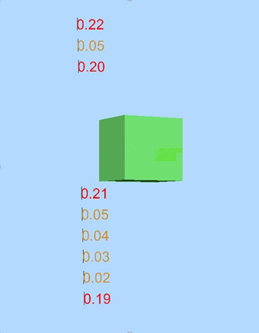Note: Sample weights shown coloured by value

If the block with results shown in Table 3 is re-estimated using 20 samples with a maximum of 12 samples per hole with an octant search requiring at least three octants, the estimated grade changes to 0.33 from 0.27. Figure 7 shows the weights and distance to sample values when using 20 samples with a maximum of 12 samples per hole with and without octants. It shows only two holes are used without octant and four where octants are used. The highest weight is still located at the end of one hole but with very similar weights for the remaining samples for each separate hole.

Figure 6 Weights with and without octants (20 samples with maximum of 12 samples per hole)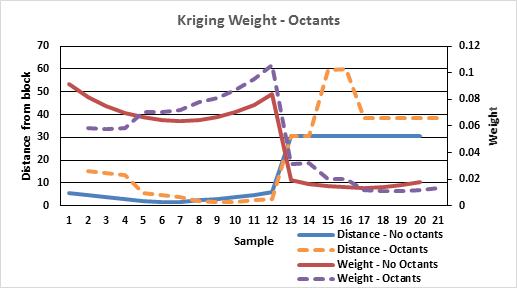Note: Step in “Distance from block” line (coloured blue and orange) is due to selection of another hole

Impact of negative weights and twinned holes: Allowing negative weights can make a great difference to the sample weights in some cases. Figure 9 shows an example of using twin holes where the drillhole that was a small distance further from the block has mainly negative weights. If you do not allow negative weights (Figure 10), samples where the weights are negative are amended to zero. A zero weight lowers the weight applied to the other samples including the greater weights applied to samples at the ends, changing the block grade.

This example shows that the use of twinned holes in block grade estimation should be avoided.

Figure 7 Negative weights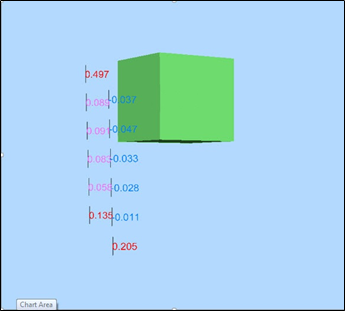Note: The sample weights are coloured by value

Figure 8 Negative weights set to zero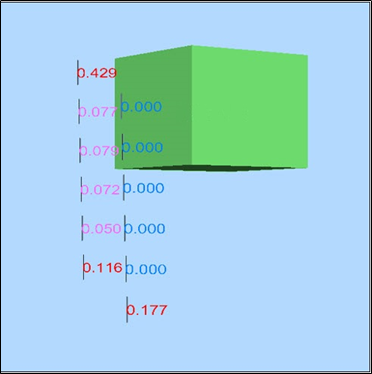Note: The values are weights coloured by values

Conclusion

These examples illustrate that when using OK for resource estimation it would be prudent to:

• Composite drillhole samples to a reasonable length, possibly approximating half the block height.
• Limit the number of samples from each hole to two or three.
• Set the maximum number of samples to allow six to ten holes to be included in the estimate.
• Include octant search.
• Allow negative weights.
• Avoid using twin holes.

This study shows that OK, like most other estimation methods, simply applies weights to the samples. The selection of samples and weights is based on location. Therefore understanding the geology and its impact on the location and grade mineralization is a must.

Rod Webster, AMC Consultants

Principal Geologist

rwebster@amcconsultants.com

1626

### Theme picker« February 2020 »
MonTueWedThuFriSatSun
27282930
30/01/2020 12:30 PM - 1:30 PMWebinar: The Year Ahead in Mining & METS

3112
345
5/02/2020 12:30 PM - 1:30 PMWebinar: Making the Most of Your Membership in 2020

6789
1011
11/02/2020 12:00 PM - 2:00 PMLocation: Ashurst Australia, 123 St Georges Terrace, PERTH, WA, 6000, AUSTRALIA

121314
14/02/2020 9:00 AM - 11:00 AMMETS Climate Change Workshop: Newcastle

Location: NIER, University of Newcastle, 70 Vale Street, Shortland, Newcastle

1516
1718
19
19/02/2020 12:30 PM - 1:30 PMWebinar: Effectively Introducing New Solutions to Mining Clients

20
20/02/2020 4:00 PM - 6:00 PMCentennial Coal Smart Mining Networking Event

Location: SMC Conference and Function Centre, 66 Goulburn Street, Sydney

212223
242526
27
28291
23456
6/03/2020 9:00 AM - 11:00 AMMETS Climate Change Workshop: Sydney

Location: SAP Office Level 6, 77 Berry Street North Sydney, NSW 2060

78

## Filter:

### Filter by Categories

GET SOCIAL